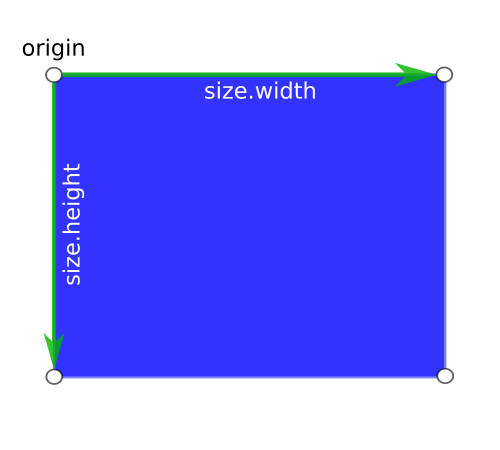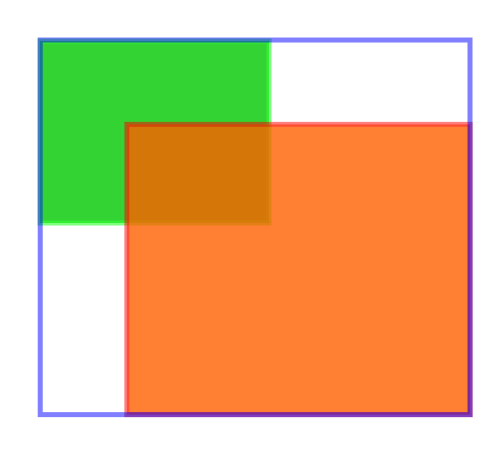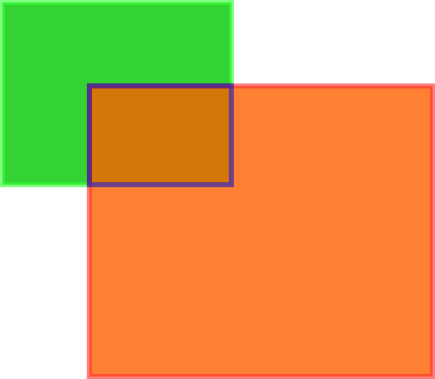## Rectangle

Rectangle — Rectangular shape type

## Functions

 #define GRAPHENE_RECT_INIT() graphene_rect_t * graphene_rect_alloc () void graphene_rect_free () graphene_rect_t * graphene_rect_init () graphene_rect_t * graphene_rect_init_from_rect () bool graphene_rect_equal () graphene_rect_t * graphene_rect_normalize () void graphene_rect_normalize_r () void graphene_rect_get_center () void graphene_rect_get_top_left () void graphene_rect_get_top_right () void graphene_rect_get_bottom_right () void graphene_rect_get_bottom_left () float graphene_rect_get_x () float graphene_rect_get_y () float graphene_rect_get_width () float graphene_rect_get_height () float graphene_rect_get_area () void graphene_rect_get_vertices () void graphene_rect_union () bool graphene_rect_intersection () bool graphene_rect_contains_point () bool graphene_rect_contains_rect () graphene_rect_t * graphene_rect_offset () void graphene_rect_offset_r () graphene_rect_t * graphene_rect_inset () void graphene_rect_inset_r () graphene_rect_t * graphene_rect_round_to_pixel () void graphene_rect_round () void graphene_rect_round_extents () void graphene_rect_expand () void graphene_rect_interpolate () const graphene_rect_t * graphene_rect_zero () void graphene_rect_scale ()

## Types and Values

 #define GRAPHENE_RECT_INIT_ZERO graphene_rect_t

## Includes

```#include <graphene.h>
```

## Description

graphene_rect_t is a type representing a rectangle through an origin graphene_point_t point and a graphene_size_t size.Operations on a graphene_rect_t will normalize the rectangle, to ensure that the origin is always the top-left corner of the rectangle and that the size is always positive.

## Functions

### GRAPHENE_RECT_INIT()

`#define             GRAPHENE_RECT_INIT(_x,_y,_w,_h)`

Initializes a graphene_rect_t when declaring it.

#### Parameters

 _x the X coordinate of the origin _y the Y coordinate of the origin _w the width _h the height

Since: 1.0

### graphene_rect_alloc ()

```graphene_rect_t *
graphene_rect_alloc (`void`);```

Allocates a new graphene_rect_t.

The contents of the returned rectangle are undefined.

#### Returns

the newly allocated rectangle.

[transfer full]

Since: 1.0

### graphene_rect_free ()

```void
graphene_rect_free (`graphene_rect_t *r`);```

Frees the resources allocated by `graphene_rect_alloc()`.

 r

Since: 1.0

### graphene_rect_init ()

```graphene_rect_t *
graphene_rect_init (`graphene_rect_t *r`,
`float x`,
`float y`,
`float width`,
`float height`);```

Initializes the given graphene_rect_t with the given values.

This function will implicitly normalize the graphene_rect_t before returning.

#### Parameters

 r x the X coordinate of the `graphene_rect_t.origin` y the Y coordinate of the `graphene_rect_t.origin` width the width of the `graphene_rect_t.size` height the height of the `graphene_rect_t.size`

#### Returns

the initialized rectangle.

[transfer none]

Since: 1.0

### graphene_rect_init_from_rect ()

```graphene_rect_t *
graphene_rect_init_from_rect (`graphene_rect_t *r`,
`const graphene_rect_t *src`);```

Initializes `r` using the given `src` rectangle.

This function will implicitly normalize the graphene_rect_t before returning.

 r src

#### Returns

the initialized rectangle.

[transfer none]

Since: 1.0

### graphene_rect_equal ()

```bool
graphene_rect_equal (`const graphene_rect_t *a`,
`const graphene_rect_t *b`);```

Checks whether the two given rectangle are equal.

 a b

#### Returns

`true` if the rectangles are equal

Since: 1.0

### graphene_rect_normalize ()

```graphene_rect_t *
graphene_rect_normalize (`graphene_rect_t *r`);```

Normalizes the passed rectangle.

This function ensures that the size of the rectangle is made of positive values, and that the origin is the top-left corner of the rectangle.

 r

#### Returns

the normalized rectangle.

[transfer none]

Since: 1.0

### graphene_rect_normalize_r ()

```void
graphene_rect_normalize_r (`const graphene_rect_t *r`,
`graphene_rect_t *res`);```

Normalizes the passed rectangle.

This function ensures that the size of the rectangle is made of positive values, and that the origin is in the top-left corner of the rectangle.

#### Parameters

 r res the return location for the normalized rectangle. [out caller-allocates]

Since: 1.4

### graphene_rect_get_center ()

```void
graphene_rect_get_center (`const graphene_rect_t *r`,
`graphene_point_t *p`);```

Retrieves the coordinates of the center of the given rectangle.

#### Parameters

 r p return location for a graphene_point_t. [out caller-allocates]

Since: 1.0

### graphene_rect_get_top_left ()

```void
graphene_rect_get_top_left (`const graphene_rect_t *r`,
`graphene_point_t *p`);```

Retrieves the coordinates of the top-left corner of the given rectangle.

#### Parameters

 r p return location for a graphene_point_t. [out caller-allocates]

Since: 1.0

### graphene_rect_get_top_right ()

```void
graphene_rect_get_top_right (`const graphene_rect_t *r`,
`graphene_point_t *p`);```

Retrieves the coordinates of the top-right corner of the given rectangle.

#### Parameters

 r p return location for a graphene_point_t. [out caller-allocates]

Since: 1.0

### graphene_rect_get_bottom_right ()

```void
graphene_rect_get_bottom_right (`const graphene_rect_t *r`,
`graphene_point_t *p`);```

Retrieves the coordinates of the bottom-right corner of the given rectangle.

#### Parameters

 r p return location for a graphene_point_t. [out caller-allocates]

Since: 1.0

### graphene_rect_get_bottom_left ()

```void
graphene_rect_get_bottom_left (`const graphene_rect_t *r`,
`graphene_point_t *p`);```

Retrieves the coordinates of the bottom-left corner of the given rectangle.

#### Parameters

 r p return location for a graphene_point_t. [out caller-allocates]

Since: 1.0

### graphene_rect_get_x ()

```float
graphene_rect_get_x (`const graphene_rect_t *r`);```

Retrieves the normalized X coordinate of the origin of the given rectangle.

 r

#### Returns

the normalized X coordinate of the rectangle

Since: 1.0

### graphene_rect_get_y ()

```float
graphene_rect_get_y (`const graphene_rect_t *r`);```

Retrieves the normalized Y coordinate of the origin of the given rectangle.

 r

#### Returns

the normalized Y coordinate of the rectangle

Since: 1.0

### graphene_rect_get_width ()

```float
graphene_rect_get_width (`const graphene_rect_t *r`);```

Retrieves the normalized width of the given rectangle.

 r

#### Returns

the normalized width of the rectangle

Since: 1.0

### graphene_rect_get_height ()

```float
graphene_rect_get_height (`const graphene_rect_t *r`);```

Retrieves the normalized height of the given rectangle.

 r

#### Returns

the normalized height of the rectangle

Since: 1.0

### graphene_rect_get_area ()

```float
graphene_rect_get_area (`const graphene_rect_t *r`);```

Compute the area of given normalized rectangle.

 r

#### Returns

the area of the normalized rectangle

Since: 1.10

### graphene_rect_get_vertices ()

```void
graphene_rect_get_vertices (`const graphene_rect_t *r`,
`graphene_vec2_t vertices[]`);```

Computes the four vertices of a graphene_rect_t.

#### Parameters

 r vertices return location for an array of 4 graphene_vec2_t. [out][array fixed-size=4]

Since: 1.4

### graphene_rect_union ()

```void
graphene_rect_union (`const graphene_rect_t *a`,
`const graphene_rect_t *b`,
`graphene_rect_t *res`);```

Computes the union of the two given rectangles.The union in the image above is the blue outline.

#### Parameters

 a b res return location for a graphene_rect_t. [out caller-allocates]

Since: 1.0

### graphene_rect_intersection ()

```bool
graphene_rect_intersection (`const graphene_rect_t *a`,
`const graphene_rect_t *b`,
`graphene_rect_t *res`);```

Computes the intersection of the two given rectangles.The intersection in the image above is the blue outline.

If the two rectangles do not intersect, `res` will contain a degenerate rectangle with origin in (0, 0) and a size of 0.

#### Parameters

 a b res return location for a graphene_rect_t. [out caller-allocates][optional]

#### Returns

`true` if the two rectangles intersect

Since: 1.0

### graphene_rect_contains_point ()

```bool
graphene_rect_contains_point (`const graphene_rect_t *r`,
`const graphene_point_t *p`);```

Checks whether a graphene_rect_t contains the given coordinates.

 r p

#### Returns

`true` if the rectangle contains the point

Since: 1.0

### graphene_rect_contains_rect ()

```bool
graphene_rect_contains_rect (`const graphene_rect_t *a`,
`const graphene_rect_t *b`);```

Checks whether a graphene_rect_t fully contains the given rectangle.

 a b

#### Returns

`true` if the rectangle `a` fully contains `b`

Since: 1.0

### graphene_rect_offset ()

```graphene_rect_t *
graphene_rect_offset (`graphene_rect_t *r`,
`float d_x`,
`float d_y`);```

Offsets the origin by `d_x` and `d_y` .

The size of the rectangle is unchanged.

#### Parameters

 r d_x the horizontal offset d_y the vertical offset

#### Returns

the offset rectangle.

[transfer none]

Since: 1.0

### graphene_rect_offset_r ()

```void
graphene_rect_offset_r (`const graphene_rect_t *r`,
`float d_x`,
`float d_y`,
`graphene_rect_t *res`);```

Offsets the origin of the given rectangle by `d_x` and `d_y` .

The size of the rectangle is left unchanged.

#### Parameters

 r d_x the horizontal offset d_y the vertical offset res return location for the offset rectangle. [out caller-allocates]

Since: 1.4

### graphene_rect_inset ()

```graphene_rect_t *
graphene_rect_inset (`graphene_rect_t *r`,
`float d_x`,
`float d_y`);```

Changes the given rectangle to be smaller, or larger depending on the given inset parameters.

To create an inset rectangle, use positive `d_x` or `d_y` values; to create a larger, encompassing rectangle, use negative `d_x` or `d_y` values.

The origin of the rectangle is offset by `d_x` and `d_y` , while the size is adjusted by `(2 * @d_x, 2 * @d_y)`. If `d_x` and `d_y` are positive values, the size of the rectangle is decreased; if `d_x` and `d_y` are negative values, the size of the rectangle is increased.

If the size of the resulting inset rectangle has a negative width or height then the size will be set to zero.

#### Parameters

 r d_x the horizontal inset d_y the vertical inset

#### Returns

the inset rectangle.

[transfer none]

Since: 1.0

### graphene_rect_inset_r ()

```void
graphene_rect_inset_r (`const graphene_rect_t *r`,
`float d_x`,
`float d_y`,
`graphene_rect_t *res`);```

Changes the given rectangle to be smaller, or larger depending on the given inset parameters.

To create an inset rectangle, use positive `d_x` or `d_y` values; to create a larger, encompassing rectangle, use negative `d_x` or `d_y` values.

The origin of the rectangle is offset by `d_x` and `d_y` , while the size is adjusted by `(2 * @d_x, 2 * @d_y)`. If `d_x` and `d_y` are positive values, the size of the rectangle is decreased; if `d_x` and `d_y` are negative values, the size of the rectangle is increased.

If the size of the resulting inset rectangle has a negative width or height then the size will be set to zero.

#### Parameters

 r d_x the horizontal inset d_y the vertical inset res return location for the inset rectangle. [out caller-allocates]

Since: 1.4

### graphene_rect_round_to_pixel ()

```graphene_rect_t *
graphene_rect_round_to_pixel (`graphene_rect_t *r`);```

`graphene_rect_round_to_pixel` has been deprecated since version 1.4 and should not be used in newly-written code.

Use `graphene_rect_round()` instead

Rounds the origin and the size of the given rectangle to their nearest integer values; the rounding is guaranteed to be large enough to contain the original rectangle.

 r

#### Returns

the pixel-aligned rectangle.

[transfer none]

Since: 1.0

### graphene_rect_round ()

```void
graphene_rect_round (`const graphene_rect_t *r`,
`graphene_rect_t *res`);```

`graphene_rect_round` has been deprecated since version 1.10 and should not be used in newly-written code.

Use `graphene_rect_round_extents()` instead

Rounds the origin and size of the given rectangle to their nearest integer values; the rounding is guaranteed to be large enough to have an area bigger or equal to the original rectangle, but might not fully contain its extents. Use `graphene_rect_round_extents()` in case you need to round to a rectangle that covers fully the original one.

This function is the equivalent of calling `floor` on the coordinates of the origin, and `ceil` on the size.

#### Parameters

 r res return location for the rounded rectangle. [out caller-allocates]

Since: 1.4

### graphene_rect_round_extents ()

```void
graphene_rect_round_extents (`const graphene_rect_t *r`,
`graphene_rect_t *res`);```

Rounds the origin of the given rectangle to its nearest integer value and and recompute the size so that the rectangle is large enough to contain all the conrners of the original rectangle.

This function is the equivalent of calling `floor` on the coordinates of the origin, and recomputing the size calling `ceil` on the bottom-right coordinates.

If you want to be sure that the rounded rectangle completely covers the area that was covered by the original rectangle — i.e. you want to cover the area including all its corners — this function will make sure that the size is recomputed taking into account the ceiling of the coordinates of the bottom-right corner. If the difference between the original coordinates and the coordinates of the rounded rectangle is greater than the difference between the original size and and the rounded size, then the move of the origin would not be compensated by a move in the anti-origin, leaving the corners of the original rectangle outside the rounded one.

#### Parameters

 r res return location for the rectangle with rounded extents. [out caller-allocates]

Since: 1.10

### graphene_rect_expand ()

```void
graphene_rect_expand (`const graphene_rect_t *r`,
`const graphene_point_t *p`,
`graphene_rect_t *res`);```

Expands a graphene_rect_t to contain the given graphene_point_t.

#### Parameters

 r p res return location for the expanded rectangle. [out caller-allocates]

Since: 1.4

### graphene_rect_interpolate ()

```void
graphene_rect_interpolate (`const graphene_rect_t *a`,
`const graphene_rect_t *b`,
`double factor`,
`graphene_rect_t *res`);```

Linearly interpolates the origin and size of the two given rectangles.

#### Parameters

 a b factor the linear interpolation factor res return location for the interpolated rectangle. [out caller-allocates]

Since: 1.0

### graphene_rect_zero ()

```const graphene_rect_t *
graphene_rect_zero (`void`);```

Returns a degenerate rectangle with origin fixed at (0, 0) and a size of 0, 0.

#### Returns

a fixed rectangle.

[transfer none]

Since: 1.4

### graphene_rect_scale ()

```void
graphene_rect_scale (`const graphene_rect_t *r`,
`float s_h`,
`float s_v`,
`graphene_rect_t *res`);```

Scales the size and origin of a rectangle horizontaly by `s_h` , and vertically by `s_v` . The result `res` is normalized.

#### Parameters

 r s_h horizontal scale factor s_v vertical scale factor res return location for the scaled rectangle. [out caller-allocates]

Since: 1.10

## Types and Values

### GRAPHENE_RECT_INIT_ZERO

```#define GRAPHENE_RECT_INIT_ZERO         GRAPHENE_RECT_INIT (0.f, 0.f, 0.f, 0.f)
```

Initializes a graphene_rect_t to a degenerate rectangle with an origin in (0, 0) and a size of 0.

Since: 1.10

### graphene_rect_t

```typedef struct {
graphene_point_t origin;
graphene_size_t size;
} graphene_rect_t;
```

The location and size of a rectangle region.

The width and height of a graphene_rect_t can be negative; for instance, a graphene_rect_t with an origin of [ 0, 0 ] and a size of [ 10, 10 ] is equivalent to a graphene_rect_t with an origin of [ 10, 10 ] and a size of [ -10, -10 ].

Application code can normalize rectangles using `graphene_rect_normalize()`; this function will ensure that the width and height of a rectangle are positive values. All functions taking a graphene_rect_t as an argument will internally operate on a normalized copy; all functions returning a graphene_rect_t will always return a normalized rectangle.

#### Members

 graphene_point_t `origin`; the coordinates of the origin of the rectangle graphene_size_t `size`; the size of the rectangle

Since: 1.0